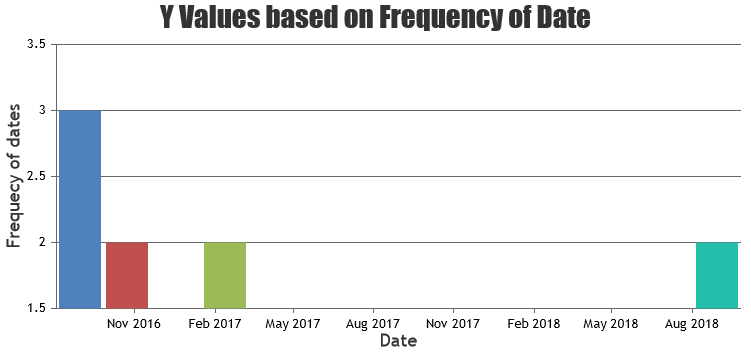Home Forums Chart Support MySQL – (Displaying Date-Time values on a Chart Axis) Reply To: MySQL – (Displaying Date-Time values on a Chart Axis)

#21961

JohnSnowGum,

You can calculate the frequency the date-occurrences in the data & build a set of datapoints. Please refer the code-snippet below.

``````function calculateFrequency(dateArray) {
dateArray.sort();
var currentXValue = null;
var count = 0;

for (var i = 0; i < dateArray.length; i++) {
if (dateArray[i] != currentXValue) {
if (count > 0)
dps.push({x: new Date(currentXValue), y: count});
currentXValue = dateArray[i];
count = 1;
} else {
count++;
}
}
if (count > 0)
dps.push({x: new Date(currentXValue), y: count});
}
``````

Please take a look at this JSFiddle for complete code. This example reads data from an array instead of database.__
Priyanka M S
Team CanvasJS